Next: Skyrme Hartree-Fock-Bogoliubov Method Up: Axially Deformed Solution of Previous: Introduction

# Hartree-Fock-Bogoliubov Method

A two-body Hamiltonian of a system of fermions can be expressed in terms of a set of annihilation and creation operators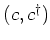: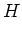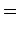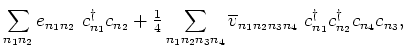(1)

where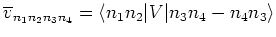are anti-symmetrized two-body interaction matrix-elements. In the HFB method, the ground-state wave function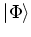is defined as the quasiparticle vacuum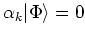, where the quasiparticle operators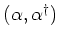are connected to the original particle operators via the linear Bogoliubov transformation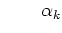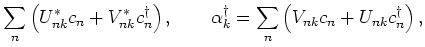(2)

which can be rewritten in the matrix form as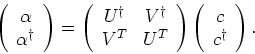(3)

Matrices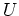and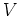satisfy the relations: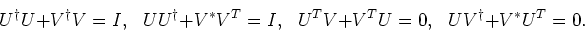(4)

In terms of the normal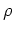and pairing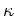one-body density matrices, defined as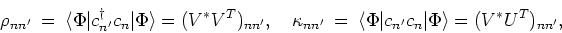(5)

the expectation value of the Hamiltonian (1) is expressed as an energy functional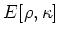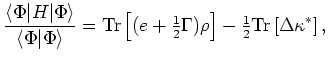(6)

where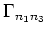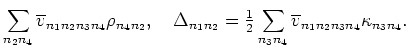(7)

Variation of energy (6) with respect toandresults in the HFB equations: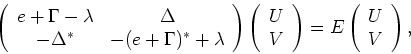(8)

where the Lagrange multiplier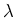has been introduced to fix the correct average particle number.

It should be stressed that the modern energy functionals (6) contain terms that cannot be simply related to some prescribed effective interaction, see e.g., Ref. [27,28] for details. In this respect the functional (6) should be considered in the broader context of the energy density functional theory.Next: Skyrme Hartree-Fock-Bogoliubov Method Up: Axially Deformed Solution of Previous: Introduction
Jacek Dobaczewski 2004-06-25